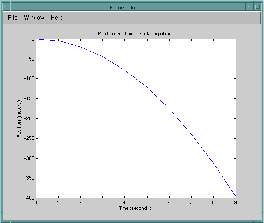## 从 C 应用程序中调用 MATLAB 函数

`matlabroot``/extern/examples/eng_mat` 文件夹中的程序 `engdemo.c` 说明如何通过独立的 C 程序调用引擎函数。此示例使用 C Matrix API`Press Return to continue`

Return 继续执行程序：

```Done for Part I. Enter a MATLAB command to evaluate. This command should create a variable X. This program will then determine what kind of variable you created. For example: X = 1:5```

```X = 17.5 X = 17.5000 Retrieving X... X is class double Done!```